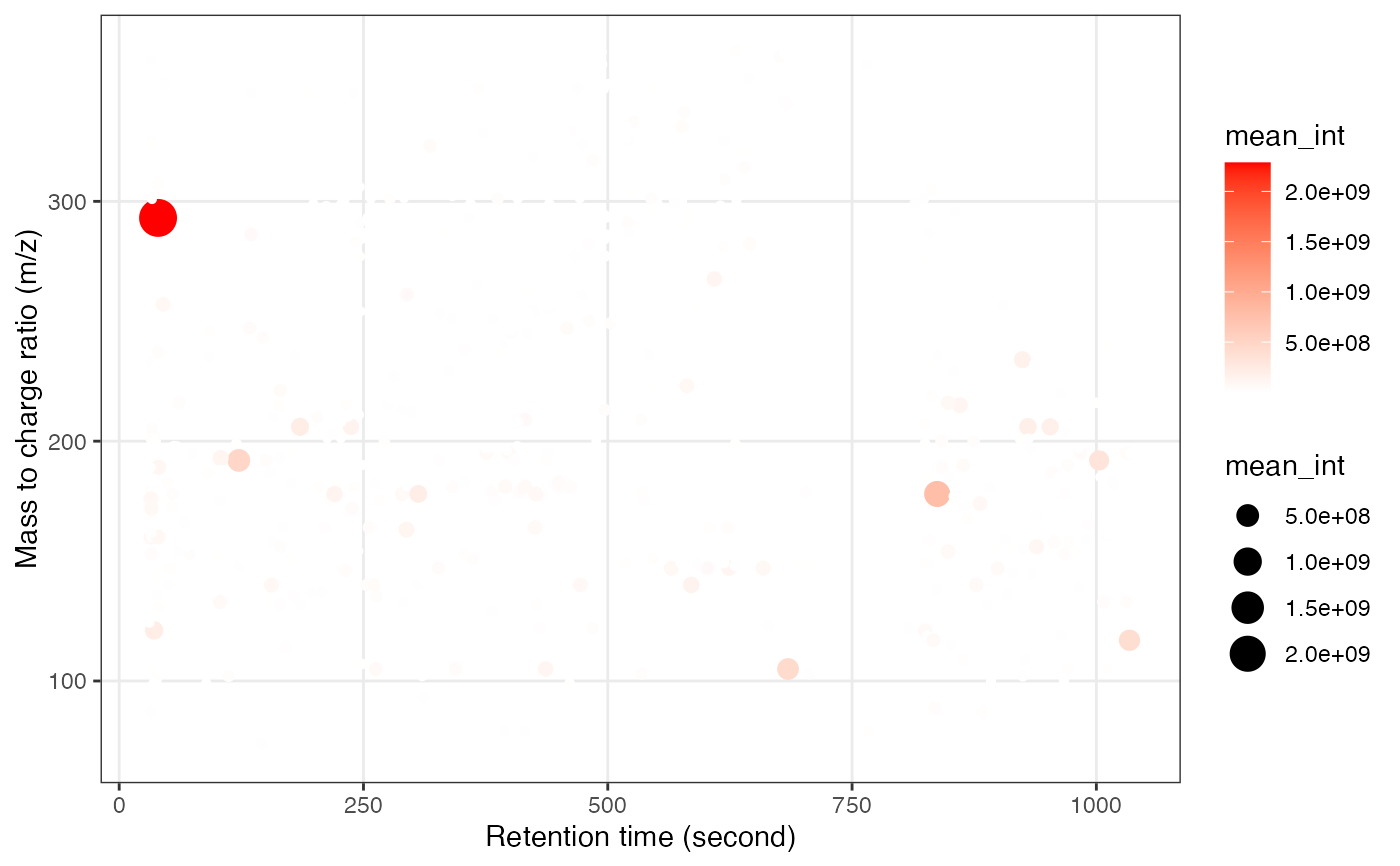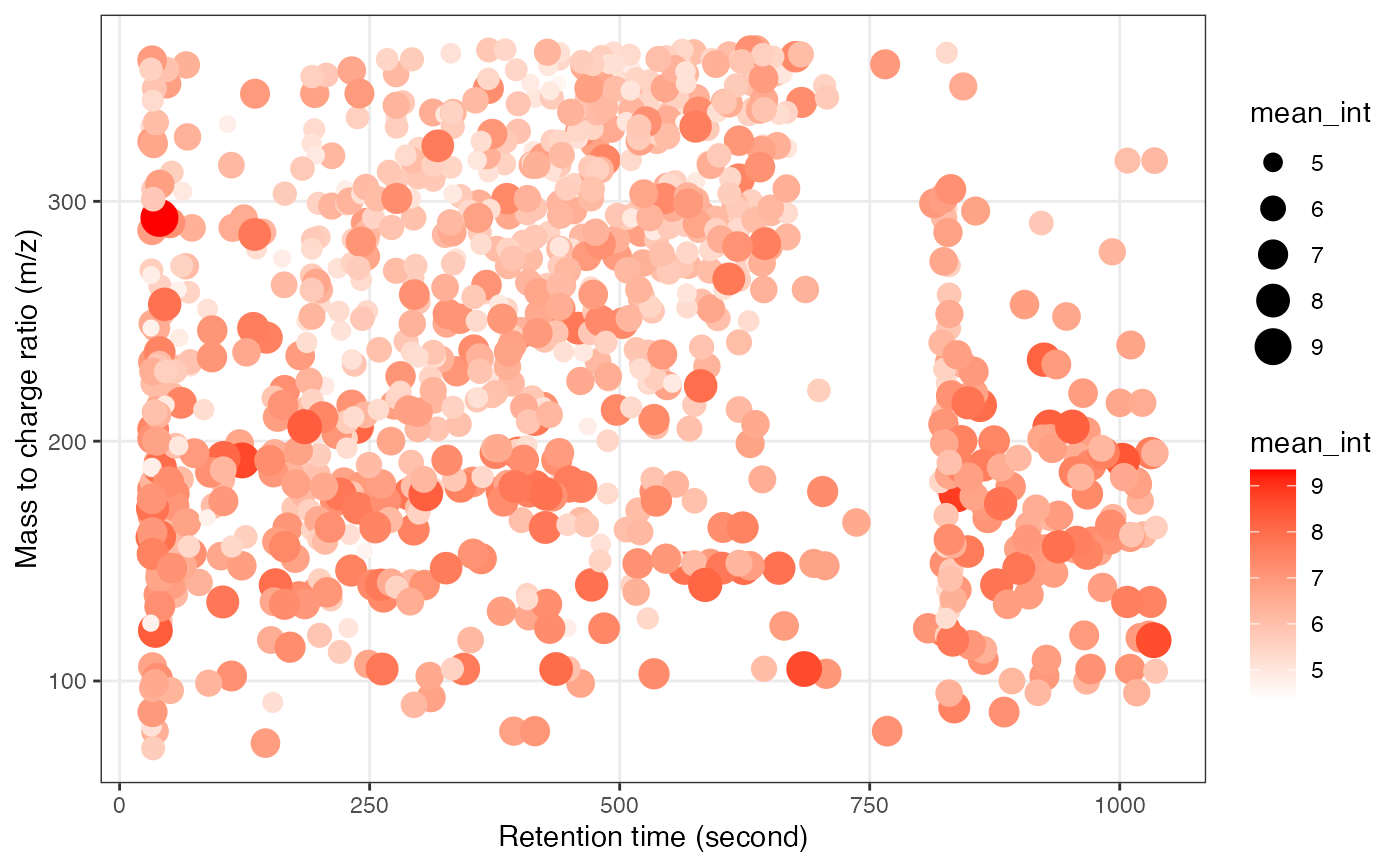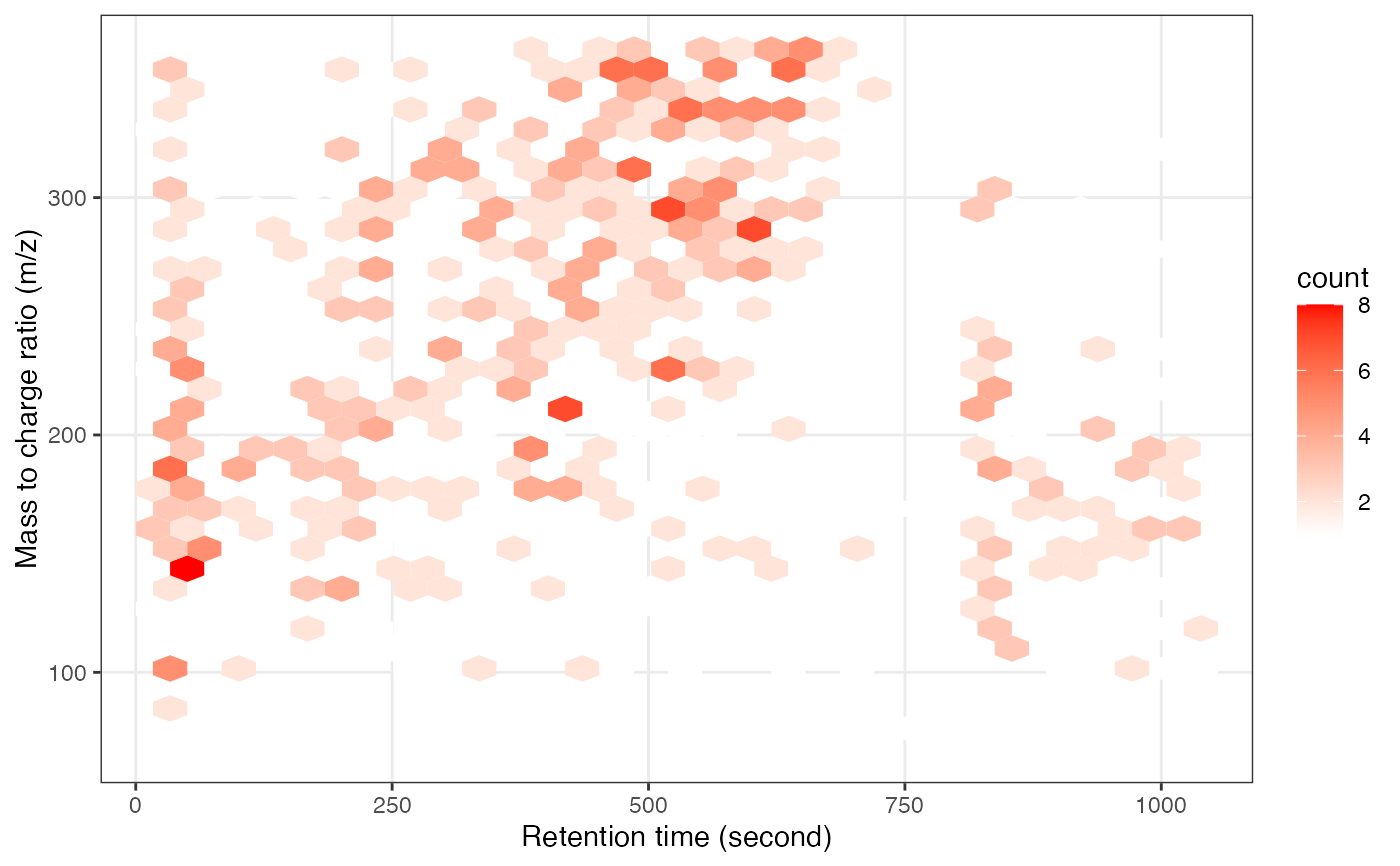This function generates a plot of mass-to-charge ratio (m/z) against retention time (rt) for a mass_dataset object. The plot can be either a hexbin plot or a scatter plot based on the hex parameter.

show_mz_rt_plot(object, hex = FALSE)

## Arguments

object

A mass_dataset object for which the mz-rt plot is to be generated.

hex

Logical. If TRUE, a hexbin plot is generated; otherwise, a scatter plot is generated. Default is FALSE.

## Value

A ggplot object representing the mz-rt plot.

## Author

Xiaotao Shen shenxt1990@outlook.com

## Examples

data("expression_data")
data("sample_info")
data("variable_info")

object =
create_mass_dataset(
expression_data = expression_data,
sample_info = sample_info,
variable_info = variable_info,
)

show_mz_rt_plot(object)show_mz_rt_plot(log(object, 10))show_mz_rt_plot(object, hex = TRUE)